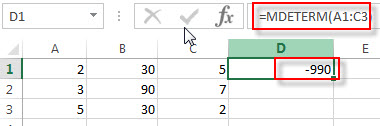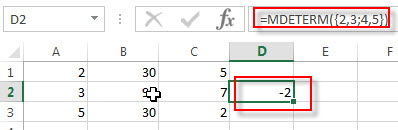# Excel MDETERM Function

This post will guide you how to use Excel MDETERM function with syntax and examples in Microsoft excel.

### Description

The Excel MDETERM function returns the matrix determinant of an array. So you can use the MDETERM function to calculate the determinant of a square matrix in Excel.

The MDETERM function is a build-in function in Microsoft Excel and it is categorized as a Math and Trigonometry Function.

The MDETERM function is available in Excel 2016, Excel 2013, Excel 2010, Excel 2007, Excel 2003, Excel XP, Excel 2000, Excel 2011 for Mac.

### Syntax

The syntax of the MDETERM function is as below:

`=MDETERM(array)`

Where the MDETERM function arguments is:

• array – This is a required argument. A numeric array with an equal number of rows and columns. So the array must provide the same number of rows and the same number of columns.

Note:

• the array can be a cell range or an array constant, such as: {2,3;5,6}
• If any cells in array are empty or contain text string, then the MDETERM function will return the #VALUE! Error.
• If the Array does not have the same number of rows and columns, then the MDETERM function will return the #VALUE! Error.
• Matrix determinants are generally used for solving systems of mathematical equations that involve several variables.
• MDETERM is calculated with an accuracy of approximately 16 digits, which may lead to a small numeric error when the calculation is not complete. For example, the determinant of a singular matrix may differ from zero by 1E-16.

### Excel MDETERM Function Examples

The below examples will show you how to use Excel MDETERM Function to calculate the determinant of a square matrix.

1# to calculate the determinant of the matrix for range A1: C3, enter the following formula in Cell D1.

`=MDETERM(A1:C3)`2# to calculate the determinant of the matrix as an array constant ({2,3;4,5}), enter the following formula in Cell D2.

`=MDETERM({2,3;4,5})`3# to calculate the determinant of the matrix as an array constant does not have an equal number of rows and columns ({2,3;4,5,6}), enter the following formula in Cell D2.

`=MDETERM({2,3;4,5,6})`

This formula returns a #VALUE! Error.

### Related Functions

• Excel MINVERSE Function
The Excel MINVERSE function returns the inverse matrix for a given matrix.The syntax of the MINVERSE function is as below:=MINVERSE(array)…
• Excel MMULT function
The Excel MMULT function returns the matrix product of two arrays.If you want to return multiple result in an array on the worksheet, then you need to input an array formula in the MMULT function.The syntax of the MMULT function is as below:=MMULT (array1,array2)…